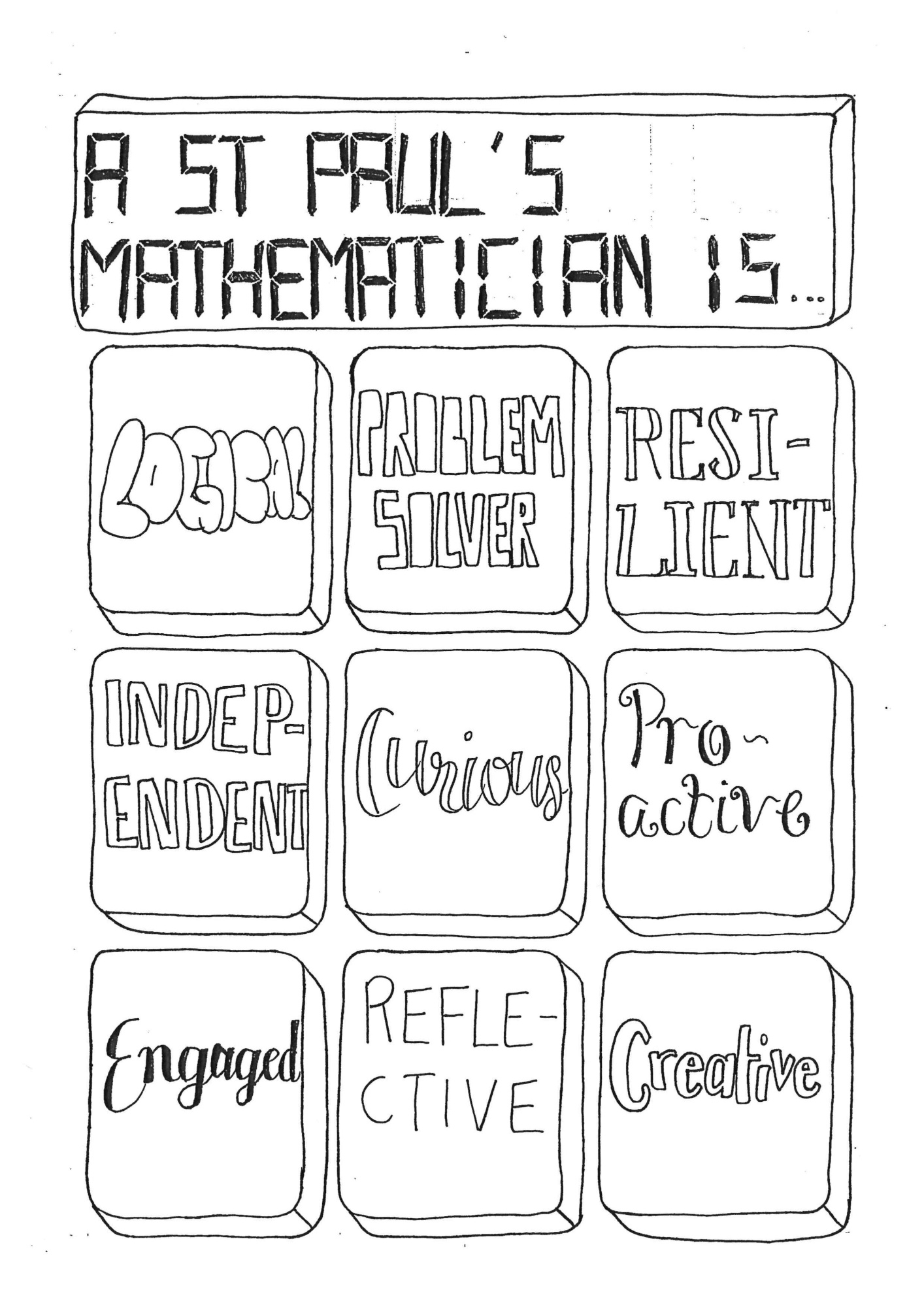# MathsThe teaching of Mathematics at St Paul’s School for Girls has the following aims to encourage students, reflecting their Key Stage, to

• develop their understanding of mathematics and mathematical processes in a way that promotes confidence and fosters enjoyment;
• develop abilities to reason logically and recognise incorrect reasoning, to generalise and to construct mathematical proofs;
• extend their range of mathematical skills and techniques and use them in unstructured problems;
• develop an understanding of coherence and progression in mathematics and of how different areas of mathematics can be connected;
• recognise how a situation may be represented mathematically and understand the relationship between ‘real world’ problems and standard and other mathematical models and how these can be refined and improved;
• acquire the skills needed to use technology such as calculators and computers effectively, recognise when such use may be inappropriate and be aware of limitations;
• develop an awareness of the relevance of mathematics to other fields of study, to the world of work and to society in general;
• take increasing responsibility for their own learning and the evaluation of their own mathematical development.

Please see these sections for further details of the maths curriculum...

Core Maths

Further Maths

KS3, KS4 and KS5